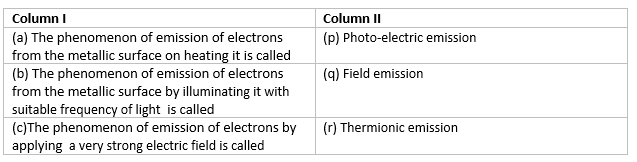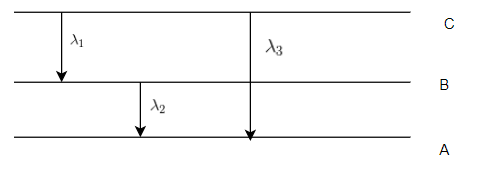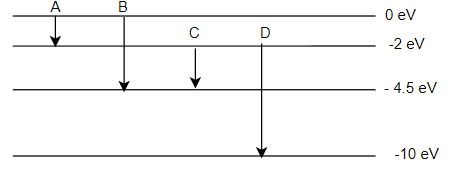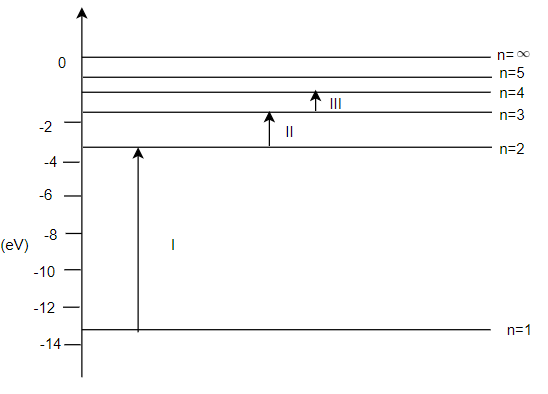# Atoms Important Questions for Class 12 Physics

## Multiple Choice Questions

Question 1
What is the angular momentum of an electron in the third orbit of an atom?
(a) $3.15 \times 10^{-34}$ Js
(b) $3.15 \times 10^{-30}$ Js
(c) $3.15 \times 10^{-31}$ Js
(d) $3.15 \times 10^{-33}$ Js

Question 2
Which series of the hydrogen spectrum has wavelength in the visible range
(a) Lyman.
(b) Balmer.
(c) Paschen.
(d) Bracket.

Question 3
The value of fine structure constant is ?
(a) $\frac {1}{136}$
(b) $\frac {1}{130}$
(c) $\frac {1}{138}$
(d) $\frac {1}{137}$

Question 4
If the electron in the hydrogen atom jumps from third orbit to second orbit, the wavelength of the emitted radiation is ?
(a) 5R/36
(b) 36/5R
(c) R/6
(d) 5/R

Question 5
if an atom moves from 2E energy level to E energy level,the wavelength $\lambda$ is emitted. If the transition takes place from 4E/3 energy level to E energy level, the wavelength emitted will be
(A) $\frac {\lambda}{3}$
(B) $3 \lambda$
(C) $\frac {3\lambda}{4}$
(D) $\frac {4\lambda}{3}$

Question 6
Maximum frequency of the emission is obtained for the transition
(a) n=2 to n=1
(b) n=6 to n=2
(c) n=1 to n=2
(d) n=2 to n=6

Question 7
The radius of an electron orbit in a hydrogen atom is of the order of
(a) $10^{-8}$ m.
(b) $10^{-9}$ m.
(c) $10^{-11}$ m.
(d) $10^{-13}$ m.

Question 8
If an electron jumps from Ist orbit to 4th Orbit then it will
(a) not lose energy.
(b) absorb energy.
(c) release energy.
(d) increases and decreases periodically

Question 9
The energy of a hydrogen atom in the ground state is -13.6 eV. The energy of a He+ ion in the first excited state will be
(a)-13.6 eV
(b)-6.8 eV
(c)-54.4.6 eV
(d)-27.2 eV

Question 10
Energy of an electron in the second orbit of hydrogen atom is E and energy of electron in 3rd orbit($E_3$) of He+ will be
(a) $E_3 = \frac {16E}{3}$
(b) $E_3 = \frac {16E}{9}$
(c) $E_3 = \frac {4E}{9}$
(d) $E_3 = \frac {4E}{3}$

Question 11
The ratio of minimum to maximum wavelength in balmer series is
(a) 1 :4.
(b) 5 : 9
(c) 3 :4
(d) 5 : 36

Question 12
The short wavelength limits of the lyman, Paschen and Balmer series in the hydrogen spectrum are a , b and c respectively . Arrange these wavelength in the increasing order?
(a)a < b < c
(b)c < b < a
(c)a < c < b
(d)b < c < a

Question 13
With increasing quantum number, the energy difference between adjacent level
(a) increases
(b) decreases
(c) same
(d) increase and then decreases

Question 14
Bohr's Radius ($r_0$) is given by?
(a) $r_0 = \frac {h^2}{8 \pi ^2 mke^2}$
(b) $r_0 = \frac {h^2}{9 \pi ^2 mke^2}$
(c) $r_0 = \frac {h^2}{2 \pi ^2 mke^2}$
(d) $r_0 = \frac {h^2}{4 \pi ^2 mke^2}$

Question 15(a) a -> p, b -> q , c -> r.
(b) a -> r, b -> q , c -> p.
(c) a -> r, b -> p , c -> q.
(d) a -> p, b -> r , c -> q.

Question 16
The ground state energy of the hydrogen atom is $E_0$. The kinetic energy of the electron in the 3rd excitel level
(a) $-\frac {E_0}{16}$
(b) $-\frac {E_0}{9}$
(c) $\frac {E_0}{16}$
(d) $\frac {E_0}{9}$

Question 17
The ground state energy of the hydrogen atom is $E_0$. The Potential energy of the electron in the 2nd excitel level
(a) $\frac {E_0}{8}$
(b) $\frac {E_0}{9}$
(c) $\frac {E_0}{16}$
(d) $\frac {2E_0}{9}$

Question 18
The electron in hydrogen atom is initially in the third excited state.What is the maximum number of lines of spectrum lines which can be emitted whne it finally moves to the ground state
(a) 3
(b) 4
(c) 5
(d)6

Question 19
A set of atoms in an excited state decays.
(a) in general to any of the states with lower energy.
(b) into a lower state only when excited by an external electric field.
(c) all together simultaneously into a lower state.
(d) to emit photons only when they collide

Question 20
A hydrogen atom and a Li++ ion are both in the second excited state. If $I_H$ and $I_{Li}$ are their respective electronic angular momentum and $E_H$ and $E_{Li}$ their respective energies,then
(a) $I_H=I_{Li}$ , $|E_{Li}| > |E_H|$
(b) $I_H < I_{Li}$ , $|E_{Li}| < |E_H|$
(c) $I_H > I_{Li}$ , $|E_{Li}| > |E_H|$
(d) $I_H=I_{Li}$ , $|E_{Li}| < |E_H|$

## Text Based Questions(long)

Question 1
Define the distance of closest approach. An alpha-particle of kinetic energy 'K' is bombarded on a thin gold foil. The distance of the closest approach is 'r'. What will be the distance of closest approach for an alpha-particle of double the kinetic energy?

Question 2
Using Bohr's postulates, obtain the expression for the total energy of the electron in the stationary states of the hydrogen atom. Hence draw the energy level diagram showing how the line spectra corresponding to Ballmer series occur due to transition between energy levels.

Question 3
(i)State Bohr's postulates to define stable orbits in hydrogen atom. How does de -broglie hypothesis explain the stability of these orbits?
(ii) A hydrogen atom initially in the ground state absorbs a photon which excites it to the n=4 level . Estimate the frequency of the photon

Question 4
Derive the Bohr's Quantisation condition for angular momentum of the orbitting of electron in hydrogen atom using de Broglie hypothesis.

Question 5
Using Bohr's postulates of the atomic model, derive the expression for the radius of the nth electron orbit.Also obtain the expression for Bohr's Radius. Show graphically the variation of the radius of the orbit with the principle quantum number

Question 1
Calculate the ratio of the frequencies of the radiation emitted due to transition of the electron in a hydrogen atom from its (i) second permitted energy level to the first level and (ii) highest permitted energy level to the second permitted level

Question 2
Calculate the shortest wavelength of light emitted in the Paschen series of hydrogen spectrum. Which part of the electromagnetic spectrum , does it belong?br> Explain the above observations on the basis of Einstein's photoelectric equation.?

Question 3
A 12.5 eV electron beam is used to excite a gaseous hydrogen atom at room temperature. Determine the wavelength and the corresponding series of the lines emmitted

Question 4
Find the relation between the three wavelength $\lambda _1$,$\lambda _2$ and $\lambda _3$ from energy level diagram shown below?Question 5
Find out the wavelength of the electron orbiting in the ground state of the hydrogen atom?

Question 6
Draw the energy-level diagram of hydrogen atom. This atom in its ground state is excited by absorption of radiation of wavelength 970 $A^o$. How many different kinds of lines are possible in the emitting spectrum?. calculate the longest wavelength among them.Take ionization energy of hydrogen atom as 13.6 eV, h=$6.6 \times 10^{-34}$ Js. c = $3 \times 10^8$ m/s ,1 eV = $1.6 \times 10^{-19}$ J

## Numerical Questions

Question 1
(i)The energy levels of an atom are as shown below. Which of them will result in the transition of a photon of wavelength 275 nm?(ii)which transition correspond to emission of radiation of maximum wavelength?
Question 2
In a Geiger Marsden experiment, calculate the distance of closest approach to the nucleus of Z=80, when an alpha-particle of 8MeV energy impings on it before it comes momentarily to rest and reverses its direction.
How will the distance of closest approach be affected when the kinetic energy of the a- particle is doubled ?

Question 3
The energy of the electron, the hydrogen atom, is known to be expressible in the form
$E_{nth}=- \frac {13.6}{n^2}$ eV n=1,2,3...
Use this expression to show that the
(i) electron in the hydrogen atom can not have an energy of -6.8 eV.
(ii) spacing between the lines (consecutive energy levels) within the given set of the observed hydrogen atom spectrum decreases as n increases.

Question 4
Photons, with a continuous range of frequencies, are made to pass through a simple of rarefied hydrogen. The transitions, shown in Fig. indicate three of the spectral absorption lines in the continuous spectrum.(i) Identify the spectral series, of the hydrogen emission spectrum, to which each of these three lines correspond.
(ii) Which of these lines corresponds to the absorption of radiation of maximum wavelength?

Question 5
Using the Rydberg formula, calculate the wavelengths of the first four spectral lines in the Lyman series of the hydrogen spectrum.
Given $h=6.6 \times 10^{-34}$ Js,$c=3 \times 10^{8}$ m/s,$e=1.6 \times 10^{-19}$ C

Question 6
A particle of charge equal to that of electron and mass 208 times the mass of electron ($\mu$ meson)moves in a circular orbit around a nucleus of charge +3e (assume mass of nucleus to be infinite). Assuming that the Bohr atom model is applicable to this system
(a) derive an expression for the radius of the nth Bohr orbit
(b) find the value of n for which the radius of the orbit is approximately the same as that of the first Bohr orbit for the hydrogen atom
(c) find the wavelength of the radiation emitted when  -meson jumps from the third orbit to the first orbit (Rydberg constant = 1.097 x 107 m-1)
Ans
(a)$r=\frac {n^2 h^2 \epsilon _0}{624 \pi m e^2}$
(b)n=25
(c)$5.478 \times 10^{-11}$ m

Question 6
Suppose that the potential energy between an electron and a proton at a distance r is given by $-\frac {ke^2}{3r^3}$ . Use Bohr theory to obtain energy level of such a hypothetical hydrogen atom.